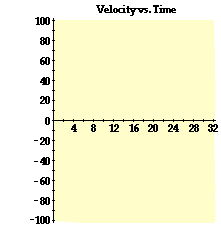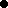### Background and Purpose:

In this activity, you will analyze the motion of a two-stage rocket. The two-stage rocket has two separate fuel stages which provide a different acceleration. After the second fuel stage is burned out, the rocket is under the sole influence of the force of gravity. Like a Fourth of July fireworks rocket, it is pre-programmed to explode at a specific time.

The purpose of this activity is to apply the principles of the graphical description of motion in order to graphically analyze the motion of the two-stage rocket. Once you have completed the activity, you have the option to take a short online quiz which targets your understanding of position-time and velocity-time graphs. The quiz will be related to the questions shown below.

### Procedure and Questions:

1. Navigate to the Two Stage Rocket Animation and experiment with the on-screen buttons in order to gain familiarity with the control of the animation. The animation can be started, paused, continued, single-stepped or rewound. Coordinate points on the graphs are displayed as the mouse is rolled over it.

After gaining familiarity with the program, use it to answer the following questions:

2. State the time interval for the various stages of the rocket's motion.

Stage 1: _________ sec to _________ sec

Stage 2: _________ sec to _________ sec

No Fuel: _________ sec to _________ sec

Rocket explodes at _________ sec

### Conceptual Analysis:

3. In what direction (up or down) is the rocket moving at each of the following times?

 t = 3.0 sec _________ t = 10.0 sec _________ t = 18.0 sec _________ t = 25.0 sec _________

4. In what direction (up or down) is the rocket accelerating at each of the following times?

 t = 3.0 sec _________ t = 10.0 sec _________ t = 18.0 sec _________ t = 25.0 sec _________

5. Is the rocket ever moving in one direction and accelerating in the opposite direction? _________

If No, then explain why it never does.
If Yes, then this occurs when the rocket is...

a. moving upward during stage 1 and stage 2.

b. moving upward after stage 2 has ended.

c. moving downward after stage 2 has ended.

d. moving (both upward and downward) after stage 2 has ended.

6. How does the acceleration of the rocket during stage 1 compare to the acceleration during stage 2?

a. The acceleration is greatest during stage 1.

b. The acceleration is greatest during stage 2.

c. The acceleration are the same.

d. The acceleration varies during the stage and it is impossible to answer.

7. How does the velocity of the rocket during stage 1 compare to the velocity during stage 2?

a. The velocity is greatest during stage 1.

b. The velocity is greatest during stage 2.

c. The velocity are the same.

d. The velocity varies during the stage and it is impossible to answer.

8. What statement best describes the changes observed of the rocket and its velocity vector after the second stage ends and there is no fuel left to power the rocket?

a. The rocket changes direction and its velocity vector continually decreases in magnitude.

b. The rocket moves upward, then changes direction; the velocity continually decreases in magnitude.

c. The rocket moves upward, then changes direction; the velocity first increases and then decreases in magnitude.

d. The rocket moves upward, then changes direction; the velocity first decreases and then increases in magnitude.

9. At what time does the rocket change its direction?

t = _________ sec

10. What is the acceleration value of the rocket when it is at its peak height? _________ m/s/s

11. What is the velocity value of the rocket when it is at its peak height? _________ m/s

12. Observe the acceleration of the rocket during the no-fuel stage of its motion. Is it constant or changing? ____________ What is its direction? ____________ What is its magnitude (i.e., numerical value)? ____________

### Graphical/Mathematical Analysis:13. A velocity-time graph is shown at the right. Sketch the graph for your rocket's motion (as displayed on the screen). Be accurate and show strategic coordinates - for example, at the end of stage 1 and stage 2 and at the rocket's peak position. These coordinates can be read off the screen by moving your mouse over the graphical display.

End of stage 1: (t, v) = ____________

End of stage 2: (t, v) = ____________

When crossing axis: (t, v) = ____________

At explosion time: (t, v) = ____________

14. On the graph at the right, put a bold dot () at the location that the rocket changes its direction. Clearly label the dot with the words "Peak". (This is a question similar to #9.)

15. Use coordinates which you listed above to calculate the slopes of the lines on the graph during stage 1, stage 2 and the no fuel stage. Show your work clearly.

 Stage 1: Stage 2: No Fuel:

16. Compare the slopes of the lines on the velocity vs. time plot (as calculated above) to the acceleration of the rocket.

 Accel'n Values: Slopes of v-t Graph: Stage 1: Stage 2: No Fuel:

What do you suppose the slope of a line on a velocity-time plot represents?

17. Use coordinates which you listed above to calculate the area between the lines on the graph and the time axis during stage 1, stage 2 and the no fuel stage (both while rising and while falling). Show your work clearly.

 Stage 1: A1 = Stage 2: A2 = No Fuel (rising): A3 = No Fuel (falling): A4 =

18. Compare the cumulative areas at the end of the various phases of motion to the heights of the rocket at the corresponding times. If necessary, re-run the animation and Single Step to the end of each stage or roll the mouse over the position-time plot to obtain height values.

 Height (m) Cumulative Area End of Stage 1: A1 = End of Stage 2: A1 + A2 = At the peak of motion: A1 + A2 + A3 = At the time of explosion: A1 + A2 + A3 + A4 =

What do you suppose the area between the line and the time axis on a velocity-time plot represents?

### Online Quiz Option:

If your teacher has instructed you to take the online quiz, then click on the Take Quiz button. The quiz questions will be directly related to the questions given above.